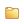# State Equations

State Equations are used to describe the thermodynamic equation relating material state variables under a given set of physical conditions, such as pressure, volume, temperature, or internal energy. State Equations are useful in describing the properties of fluids, mixtures of fluids, and so on.State Equation entities are only available in the LS-DYNA profiles.

A State Equation entity is created on import of an input deck containing at least one of the supported cards listed below.

State Equations have an active and export state. The active state of a State Equation entity controls its listing in the Model Browser and any of its views. The export state of a State Equation entity controls whether or not this entity is exported when the custom export option is used. The “all” export option is not affected by the export state. The active and export states of State Equation entities can be controlled using the Entity State Browser.

## LS-DYNA Cards

• *EOS_GRUNEISEN
• *EOS_IDEAL_GAS
• *EOS_IGNITION_AND_GROWTH_OF_REACTION_IN_HE
• *EOS_JWL
• *EOS_LINEAR_POLYNOMIAL
• *EOS_LINEAR_POLYNOMIAL_WITH_ENERGY_LEAK
• *EOS_PROPELLANT_DEFLAGRATION
• *EOS_RATIO_OF_POLYNOMIALS
• *EOS_SACK_TUESDAY
• *EOS_TABULATED
• *EOS_TABULATED_COMPACTION
• *EOS_TENSOR_PORE_COLLAPSE